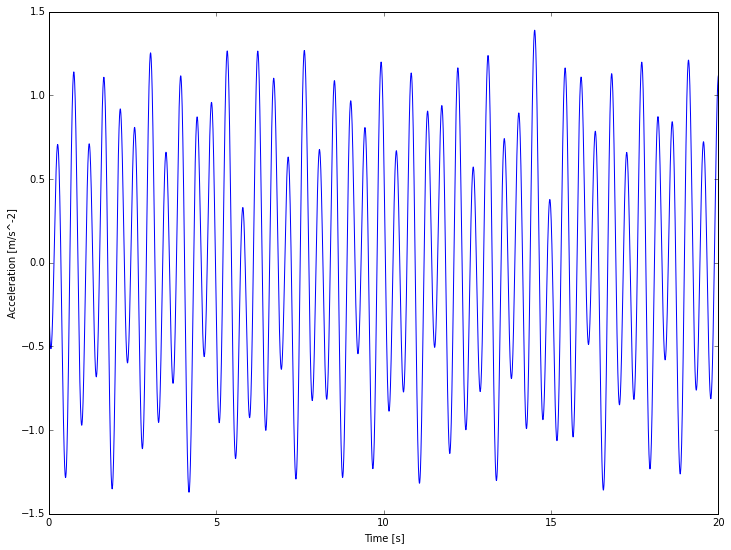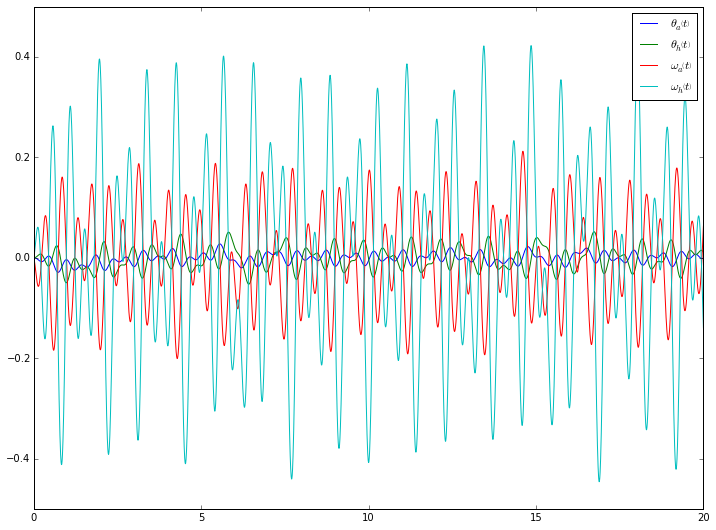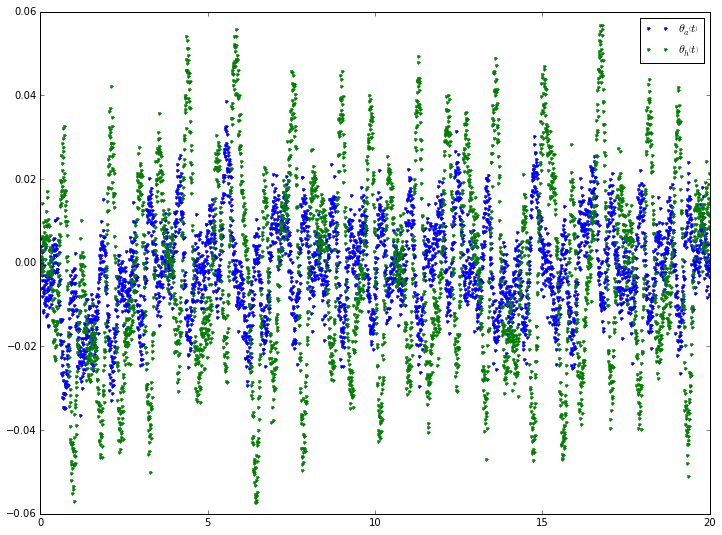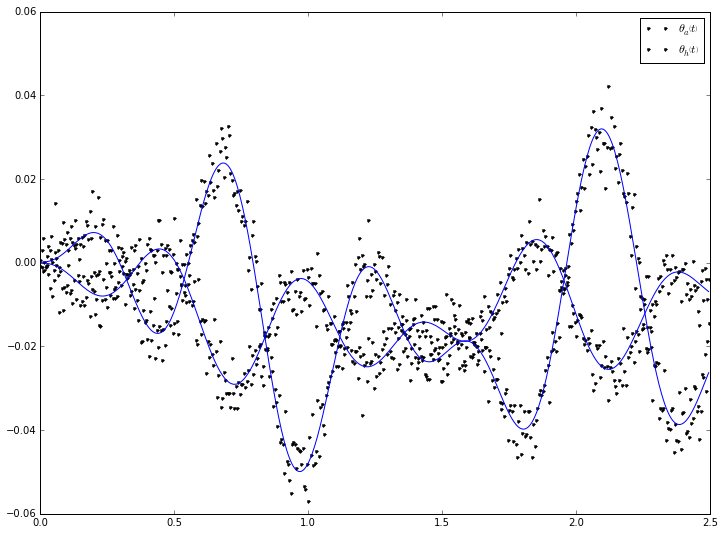# Introduction¶

This problem mirrors the identification of the control gains used in standing from:

Park, Sukyung, Fay B. Horak, and Arthur D. Kuo. "Postural Feedback Responses Scale with Biomechanical Constraints in Human Standing." Experimental Brain Research 154, no. 4 (February 1, 2004): 417-27. doi:10.1007/s00221-003-1674-3.

except that we identify from much longer times series and use methods that are order's of magnitude faster. The model is shown in the figure below:# Imports¶

In :
import numpy as np
import sympy as sm
import sympy.physics.mechanics as me
from scipy.integrate import odeint
import matplotlib.pyplot as plt
from IPython.core.pylabtools import figsize
from opty.direct_collocation import Problem
from opty.utils import sum_of_sines, parse_free

from model import PlanarStandingHumanOnMovingPlatform


The following will all the symbolics to be rendered correctly.

In :
me.init_vprinting(use_latex='mathjax')


This causes the matplotlib plots to show inline in the notebook and sets the default figure size.

In :
%matplotlib inline
figsize(12.0, 9.0)


# Derive the symbolic model¶

The model is derived using PyDy and SymPy mechanics. This class exercises the APIs behind the scene to generate the equations of motion of the system. This model also has scaling feature that is useful for good convergence of the NLP problem.

In :
model = PlanarStandingHumanOnMovingPlatform(unscaled_gain=0.5)
model.derive()


The model has four states, an angle and angular rate for the ankle and hip.

In :
model.states()

Out:
$$\left [ \theta_{a}, \quad \theta_{h}, \quad \omega_{a}, \quad \omega_{h}\right ]$$

The model constants are shown below. The $s$ terms are the scaled gains.

In :
model.closed_loop_par_map

Out:
$$\left \{ I_{L} : 1.79917382349, \quad I_{T} : 2.48116350633, \quad d_{L} : 0.571598315274, \quad d_{T} : 0.314491823529, \quad g : 9.81, \quad l_{L} : 0.878, \quad m_{L} : 32.125955184, \quad m_{T} : 48.8305665951, \quad s_{00} : 1900.0, \quad s_{01} : 350.0, \quad s_{02} : 370.0, \quad s_{03} : 100.0, \quad s_{10} : 90.0, \quad s_{11} : 580.0, \quad s_{12} : 120.0, \quad s_{13} : 52.0\right \}$$

The full state feedback matrix has this form:

In :
model.gain_matrix

Out:
$$\left[\begin{matrix}k_{00} & k_{01} & k_{02} & k_{03}\\k_{10} & k_{11} & k_{12} & k_{13}\end{matrix}\right]$$

Finally the implicit form of the equations of motion can be output:

In :
model.first_order_implicit()

Out:
$$\left[\begin{matrix}\omega_{a} - \dot{\theta}_{a}\\\omega_{h} - \dot{\theta}_{h}\\d_{L} g m_{L} \operatorname{sin}\left(\theta_{a}\right) + d_{L} m_{L} a \operatorname{cos}\left(\theta_{a}\right) + d_{T} g m_{T} \operatorname{sin}\left(\theta_{a} + \theta_{h}\right) + d_{T} l_{L} m_{T} \left(\omega_{a} + \omega_{h}\right)^{2} \operatorname{sin}\left(\theta_{h}\right) - d_{T} l_{L} m_{T} \omega^{2}_{a} \operatorname{sin}\left(\theta_{h}\right) + d_{T} m_{T} a \operatorname{cos}\left(\theta_{a} + \theta_{h}\right) + g l_{L} m_{T} \operatorname{sin}\left(\theta_{a}\right) - k_{00} s_{00} \theta_{a} - k_{01} s_{01} \theta_{h} - k_{02} s_{02} \omega_{a} - k_{03} s_{03} \omega_{h} + l_{L} m_{T} a \operatorname{cos}\left(\theta_{a}\right) - \left(I_{T} + d_{T} m_{T} \left(d_{T} + l_{L} \operatorname{cos}\left(\theta_{h}\right)\right)\right) \dot{\omega}_{h} - \left(I_{L} + I_{T} + d_{L}^{2} m_{L} + m_{T} \left(d_{T}^{2} + 2 d_{T} l_{L} \operatorname{cos}\left(\theta_{h}\right) + l_{L}^{2}\right)\right) \dot{\omega}_{a}\\d_{T} g m_{T} \operatorname{sin}\left(\theta_{a} + \theta_{h}\right) - d_{T} l_{L} m_{T} \omega^{2}_{a} \operatorname{sin}\left(\theta_{h}\right) + d_{T} m_{T} a \operatorname{cos}\left(\theta_{a} + \theta_{h}\right) - k_{10} s_{10} \theta_{a} - k_{11} s_{11} \theta_{h} - k_{12} s_{12} \omega_{a} - k_{13} s_{13} \omega_{h} - \left(I_{T} + d_{T}^{2} m_{T}\right) \dot{\omega}_{h} - \left(I_{T} + d_{T} m_{T} \left(d_{T} + l_{L} \operatorname{cos}\left(\theta_{h}\right)\right)\right) \dot{\omega}_{a}\end{matrix}\right]$$

# Generate "Measured" Data¶

We will generate data for a duration of 20 seconds with the measurement sampling matching the discretization of the collocation problem, both at 200 hz.

In :
num_nodes = 4000
duration = 20.0
interval = duration / (num_nodes - 1)

time = np.linspace(0.0, duration, num=num_nodes)


This model is capable of includeing reference tracking noise, but for this example we will set the reference tracking noise to zero.

In :
ref_noise = np.zeros((len(time), 4))


We will use a sum of sines to simulate a pseudo-random lateral input accelration to the base.

In :
nums = [7, 11, 16, 25, 38, 61, 103, 131, 151, 181, 313, 523]
freq = 2.0 * np.pi * np.array(nums, dtype=float) / 240.0
pos, vel, accel = sum_of_sines(0.01, freq, time)

In :
plt.plot(time, accel)
plt.xlabel('Time [s]')
plt.ylabel('Acceleration [m/s^-2]')

Out:
<matplotlib.text.Text at 0x7fc51083bf50>Now we generate a function that evaluates the right hand side of the closed loop system:

$$\dot{\mathbf{x}} = \mathbf{f}_c(\mathbf{x}, \mathbf{r}_k, \mathbf{p}_k, \mathbf{p}_u, t)$$
In :
rhs, r, p = model.closed_loop_ode_func(time, ref_noise, accel)
help(rhs)

Help on function rhs in module pydy.codegen.ode_function_generators:

rhs(*args)
Returns the derivatives of the states, i.e. numerically evaluates the right
hand side of the first order differential equation.

x' = f(x, t, r, p)

Parameters
==========
x : ndarray, shape(4,)
The state vector is ordered as such:
- theta_a(t)
- theta_h(t)
- omega_a(t)
- omega_h(t)
t : float
The current time.
r : ndarray, shape(3,)

A ndarray with the specified values in the correct order and of the
correct shape.

The specified inputs are, in order:
- a(t)
- T_h(t)
- T_a(t)
p : ndarray shape(8,)
A ndarray of floats that give the numerical values of the constants in
this order:
- l_L
- d_L
- d_T
- m_L
- m_T
- I_L
- I_T
- g

Returns
=======
dx : ndarray, shape(4,)
The derivative of the state vector.



The system can be simulated by integrating the right hand side through time, given some initial condition.

In :
x0 = np.zeros(len(model.states()))

In :
x = odeint(rhs, x0, time, args=(r, p))

In :
plt.plot(time, x)
plt.legend([sm.latex(s, mode='inline') for s in model.states()])

Out:
<matplotlib.legend.Legend at 0x7fc51088fe10>Now we add measurement noise to these simulated trajectories.

In :
accel_meas = accel + np.random.normal(scale=np.deg2rad(0.25), size=accel.shape)

In :
x_meas = x + np.random.normal(scale=np.deg2rad(0.25), size=x.shape)

In :
plt.plot(time, x_meas[:, :2], '.')
plt.legend([sm.latex(s, mode='inline') for s in model.states()])

Out:
<matplotlib.legend.Legend at 0x7fc5106bf710># Define the objective¶

$$\min\limits_\theta J(\mathbf{\theta}), \quad J(\mathbf{\theta})= \sum_{i=1}^N h [\mathbf{x}_{mi} - \mathbf{x}_i]^2$$
In :
x_meas_vec = x_meas.T.flatten()

In :
def obj(free):
return interval * np.sum((x_meas_vec - free[:4 * num_nodes])**2)

grad[:4 * num_nodes] = 2.0 * interval * (free[:4 * num_nodes] - x_meas_vec)


# Define bounds on the gains¶

In :
bounds = {}
for g in model.gain_symbols:
bounds[g] = (0.0, 1.0)
bounds

Out:
$$\left \{ k_{00} : \left ( 0.0, \quad 1.0\right ), \quad k_{01} : \left ( 0.0, \quad 1.0\right ), \quad k_{02} : \left ( 0.0, \quad 1.0\right ), \quad k_{03} : \left ( 0.0, \quad 1.0\right ), \quad k_{10} : \left ( 0.0, \quad 1.0\right ), \quad k_{11} : \left ( 0.0, \quad 1.0\right ), \quad k_{12} : \left ( 0.0, \quad 1.0\right ), \quad k_{13} : \left ( 0.0, \quad 1.0\right )\right \}$$

# Initialize the problem¶

In :
prob = Problem(obj, obj_grad,
model.first_order_implicit(),
model.states(),
num_nodes,
interval,
known_parameter_map=model.closed_loop_par_map,
known_trajectory_map={model.specified['platform_acceleration']: accel_meas},
bounds=bounds,
time_symbol=model.time,
integration_method='midpoint')


In :
prob.addOption('linear_solver', 'ma57')


The initial guess can be:

$$\mathbf{\theta}_0 = [\mathbf{x}_{m1}, \ldots, \mathbf{x}_{mN}, \mathbf{0}]$$

But it can also solve from:

$$\mathbf{\theta}_0 = \mathbf{0}$$
In :
#initial_guess = np.hstack((x_meas_vec, np.zeros(8)))
initial_guess = np.zeros(prob.num_free)


# Solve the problem¶

In :
solution, info = prob.solve(initial_guess)

In :
x_sol, r_sol, p_sol = parse_free(solution, len(model.states()), 0, num_nodes)

In :
print("Gain initial guess: {}".format(model.gain_scale_factors.flatten() * initial_guess[-8:]))

Gain initial guess: [ 0.  0.  0.  0.  0.  0.  0.  0.]

In :
print("Known value of p = {}".format(model.numerical_gains.flatten()))

Known value of p = [ 950.  175.  185.   50.   45.  290.   60.   26.]

In :
print("Identified value of p = {}".format(model.gain_scale_factors.flatten() * p_sol))

Identified value of p = [ 947.77492904  173.66745739  185.12855097   49.82707837   44.14240269
289.58708362   60.04449855   25.95846021]


# Plots¶

The trajectories of the states fit the measured data.

In :
plt.plot(time[:500], x_meas[:500, :2], '.k', time[:500], x_sol.T[:500, :2], 'b')
plt.legend([sm.latex(s, mode='inline') for s in model.states()[:2]])

Out:
<matplotlib.legend.Legend at 0x7fc5108a5110>